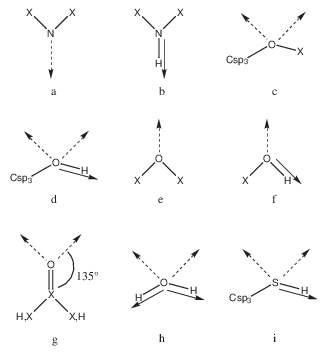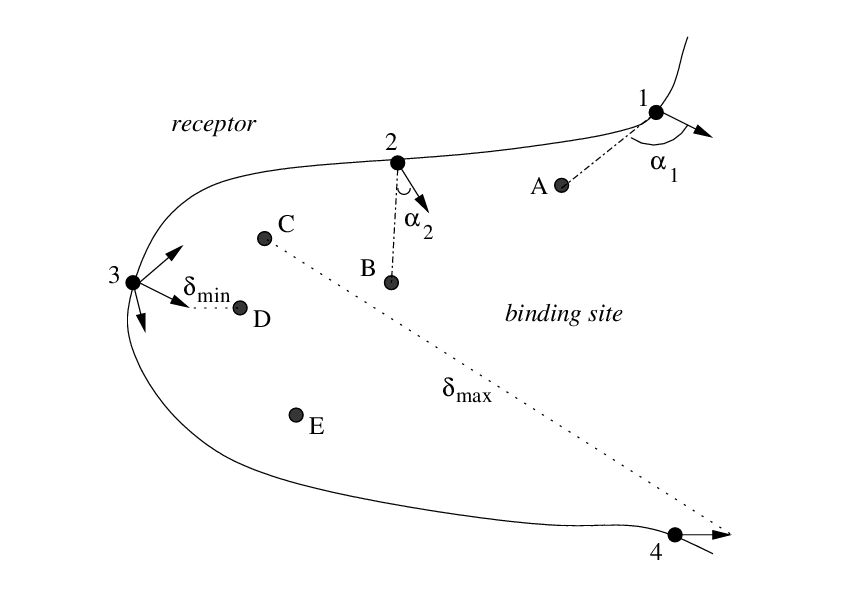# Vectors for docking¶

The binding site where the fragments are to be docked is defined by a list of receptor residues (possibly including explicit water molecules, treated as part of the receptor molecule). The first line of i3 is the number of residues in the binding site and the following lines are the residue sequential numbers (e.g. if ARG38 is the first residue of the protein, its sequential number is 1 and not 38). If a metal ion belongs to the binding site, its sequential number also has to be in the list.

## Vectors for polar docking¶

Fragments are considered polar if they have at least one H-bond donor or acceptor. SEED docks polar fragments where at least one hydrogen bond with good geometry is made.

First, predefined rules (see figure) allow the distribution of vectors of unitary length on all H-bond groups of the fragment in a direction for an ideal H-bond geometry. For example, if a nitrogen atom is bound to two heavy atoms, one H-bond vector is generated in the direction of either the lone pair (Figure 1a) or the NH bond (Figure 1b). The same procedure is then used for the polar groups in the receptor binding site (backbone and side chains). These rules are based on the atomic element number. A correspondence between atom types and atomic element numbers has to be specified in p29: the first line is the total number of correspondences and the first three terms of the following lines are respectively a sequential index number, the atom type and the atomic element number. Vectors for metal ions have to be provided by the user. The first line of i5 is the total number of vectors for the metal ions and each of the following lines contains the atom number of the metal as it is in the receptor mol2 file and the coordinates of the vector extremity. The vector is then built by joining the vector extremity to the metal ion center.Description of polar vectors for the fragment and for the receptor. X is a heavy atom. The broken arrow represents a vector of H-bond acceptor in the lone pair direction and the full arrow a vector of H-bond donor. The geometry of c, d, h and i is tetrahedral (angle of $$109^\circ$$). Examples: (a) imidazole, pyridine, (b) protein backbone, imidazole, indole, (c) ethers, (d) Ser and Thr side chains, sugars, (e) methoxybenzene, (f) Tyr side chain, phenol, (g) Asn, Gln, Asp, and Glu side chains, protein backbone, acetamide, (h) water, (i) Cys side chain.

For the receptor polar groups and metal ions an additional set of vectors is distributed uniformly on a spherical region around each of the ideal directions to increase the spatial sampling. The first term of p12 is the maximal angular deviation from ideal hydrogen bond geometry and the second term is the number of additional vectors to distribute uniformly on the spherical region.

To discard receptor vectors that point into a region of space occupied by other atoms of the protein and select preferentially vectors in the concave regions of the receptor, a spherical probe is set on the vector extremity at a distance corresponding to the sum of the van der Waals radii of the acceptor or donor atom and the probe. The van der Waals radius of the probe in Å is specified in p15 and those of the atom types are specified in the 4th column of p29. The van der Waals interaction (see below) between the probe and all the receptor atoms is then evaluated except for the receptor hydrogen atom involved in the H-bond. The vectors which show less favorable van der Waals energies are discarded. The number of selected polar vectors is modified through the first term of p2.

Finally, the docking itself is achieved by matching an H-bond vector of the receptor with an H-bond vector of the fragment at a distance that depends on the atom types of donor and acceptor involved in the hydrogen bond. These bond lengths are specified in p30 (a default length on the first line and two blocks where lengths are set between element types and atom types respectively; each block starts with the number of following lines in the block). The fragment is then rotated around the H-bond axis to increase sampling. The number of rotations is set in p13.

## Vectors for apolar docking¶

SEED docks apolar fragments into hydrophobic regions of the receptor. First, a number of points are distributed uniformly on the solvent-accessible surface (SAS) of the fragment. The density of surface points for the fragment is set in the second term of p22. Second, an automatic procedure defines the hydrophobic regions on the receptor. For this purpose a number of points are uniformly distributed on the SAS of the binding site (density of surface points for the receptor in the first term of p22). A low dielectric sphere is placed on each of these points, and the receptor desolvation energy and the probe/receptor van der Waals interaction are evaluated. The radius of the sphere is the third term of p22: a value of 1.4 Å allows a finer description of the narrow pockets than with a value of 1.8 Å. The points on the receptor SAS are then ranked according to the sum of the two energy terms weighted by scaling factors that are set by the last two terms of p22. The number of selected apolar points can be modified with the second term of p2. For both the fragment and the receptor, vectors are defined by joining each point on the SAS with the corresponding atom center. Finally, apolar fragments are docked by matching a vector of the fragment with a vector of the receptor at the optimal van der Waals distance. To improve sampling additional rotations of the fragment are performed around the axis joining the receptor atom and fragment atom. The number of rotations is set in p13.

## Angle criterion¶

To discard polar and apolar receptor vectors that point outside of the binding site a selection using an angle criterion (see figure) can be activated (i4 and p14).1-4: receptor atoms and vectors. A-E: user-defined anchor points in the binding site (e.g., fragment heavy atoms). The angle between a vector and its closest anchor point in the binding site is shown for two vectors ($$\alpha_1$$, $$\alpha_2$$). Reasonable parameters should allow to remove the vector of atom 1 from the list of receptor vectors and keep the vector of atom 2. $$\delta_{min}$$ and $$\delta_{max}$$ are defined in the text.

It is applied directly after vectors have been distributed on the binding site, i.e., before the selection by means of a spherical probe for polar vectors and before the selection by means of a low dielectric sphere for apolar vectors. The first line of i4 is the number of user-defined anchor points in the binding site and the following lines are their coordinates. The anchor points can be for example the fragment heavy atoms obtained from a known fragment-receptor complex structure. The minimal and maximal distances ($$\delta_{min}$$ and $$\delta_{max}$$) between the extremity of the vectors and the anchor points in the binding site are first evaluated. A vector is then discarded if the angle between the vector and the closest anchor point in the binding site (angle anchor_point–vector_origin–vector_extremity) is larger than an angle cutoff. The angle cutoff is p141 (first parameter in p14) if the distance between the vector and the closest anchor point is smaller or equal to $$\delta_{min} \times$$ p143; the angle cutoff is p142 if the distance is larger or equal to $$\delta_{max} \times$$ p144. For other distances the angle cutoff value falls between p141 and p142 (linear dependence). Reasonable parameters provide permissive angle cutoffs for vectors close to an anchor point and stricter angle cutoffs for distant vectors. If the choice of p14 is not appropriate, there might be cases where $$\delta_{min} \times$$ p143$$>=\delta_{max} \times$$ p144; When this happens, the more permissive angle cutoff (p141) is always used.

## Polar and apolar docking¶

Some “polar” fragments can have considerable hydrophobic character (e.g., diphenyl- ether). Therefore, they can also be docked by the procedure for apolar fragments. The second column of i7 allows the user to select apolar docking (a), polar docking (p) or both (b).